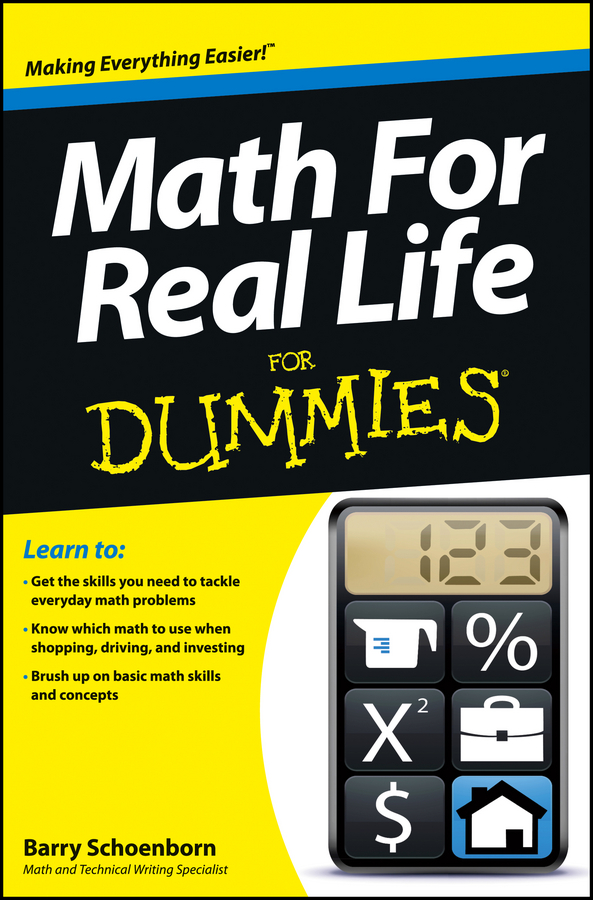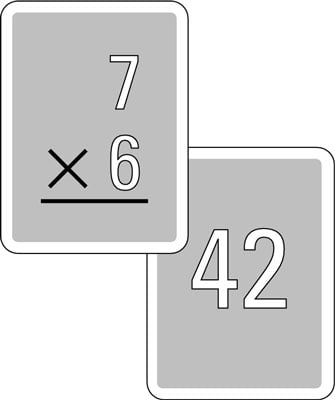##### Math For Real Life For DummiesIf you have trouble multiplying numbers, then you should get to know the multiplication table. There are two multiplication tables you can use: the cumbersome old table, and the newer, easy-to-use short multiplication table.

## The old multiplication table

One glance at the old multiplication table, shown below, reveals the problem. If you saw the movie Amadeus, you may recall that Mozart was criticized for writing music that had “too many notes.” Well, you may find that the multiplication table has too many numbers.

Just looking at it makes many people’s eyes glaze over. With 100 numbers to memorize, no wonder so many folks just give up and carry a calculator.

## The short multiplication table

If the above multiplication table were smaller and a little more manageable, you’d probably like it a lot more. So here’s a short multiplication table.

As you can see, this has a lot fewer numbers. In fact, the table is reduced from 100 numbers to 28. And 11 of the remaining numbers are bold. (You’ll see why in a moment.)

Is just slashing and burning the sacred multiplication table wise? Is it even legal? Well, of course it is! After all, the table is just a tool, like a hammer. If a hammer’s too heavy to pick up, then you ought to buy a lighter one. Similarly, if the multiplication table is too big to work with, then you need a smaller one. Besides, only the numbers you don’t need have been removed. For example, the condensed table doesn’t include rows or columns for 0, 1, or 2. Here’s why:

• Any number multiplied by 0 is 0 (people call this trait the zero property of multiplication).

• Any number multiplied by 1 is that number itself (which is why mathematicians call 1 the multiplicative identity).

• Multiplying by 2 is fairly easy; if you can count by 2s — 2, 4, 6, 8, 10, and so forth — you can multiply by 2.

The rest of the removed numbers are redundant. (And not just redundant, but also repeated, extraneous, and unnecessary!) For example, any way you slice it, 3 5 and 5 3 are both 15 (you can switch the order of the factors because multiplication is commutative). In this condensed table, the clutter has simply been removed.

So what’s left? Just the numbers you need. These numbers include a bold row and a bold diagonal. The bold row is the 5 times table, which you probably know pretty well. (In fact, they may evoke a childhood memory of running to find a hiding place on a warm spring day while one of your friends counted in a loud voice: 5, 10, 15, 20, ...)

The bold numbers on the diagonal are the square numbers. When you multiply any number by itself, the result is a square number. You probably know these numbers better than you think.

## Memorize the short multiplication table

In about an hour, you can make huge strides in memorizing the multiplication table. To start out, make a set of flash cards that give a multiplication problem on the front and the answer on the back. They may look like the following figure.Remember, you need to make only 28 flash cards — one for every example in the short table above. Split these 28 into two piles — a “bold” pile with 11 cards and a “regular” pile with 17. Then begin:

1. 5 minutes:

Work with the bold pile, going through it one card at a time. If you get the answer right, put that card on the bottom of the pile. If you get it wrong, put it in the middle so you get another chance at it more quickly.

2. 10 minutes:

Switch to the regular pile and work with it in the same way.

3. 15 minutes:

Repeat Steps 1 and 2.

Now take a break. Really — the break is important to rest your brain. Come back later in the day and do the same thing.

After you’re done with this exercise, you should find going through all 28 cards with almost no mistakes to be fairly easy. At this point, feel free to make cards for the rest of the standard times table — you know, the cards with all the 0, 1, 2 times tables on them and the redundant problems — mix all 100 cards together, and amaze your family and friends.